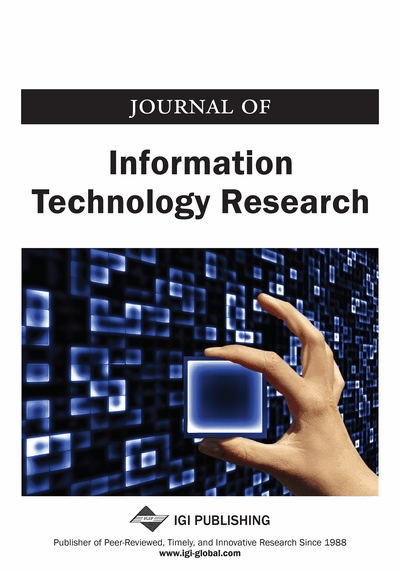# A Model Driven Engineering Approach to Reduce Large Queueing Networks

Ahlem Nasri (RELA(CS)2 Laboratory, University of Oum El Bouaghi, Oum El Bouaghi, Algeria) and Abdelhabib Bourouis (RELA(CS)2 Laboratory, University of Oum El Bouaghi, Oum El Bouaghi, Algeria)
DOI: 10.4018/JITR.2017040101

## Abstract

Modeling complex systems, including discrete event systems, remains a challenge. The complexity and the size of such systems prevent understanding their models. This article proposes an approach for reducing queuing networks large models into smaller ones. The objective is to reduce the analysis as well as the simulation times in addition to the better understanding of the system under study. The basic idea is to divide the model into a set of smaller, hierarchically organized and more manageable sub-models, which are analyzed in isolation. The key contributions of this work are the substitution of each sub-model by a single M/G/8 station and the automation of the decomposition process using model transformation techniques. The main conclusion is that the reduction approach provides exact results for the global mean number of clients and mean residence time at the whole network.
Article Preview
Top

## 1. Introduction

Queueing networks are the most known formalism used for simulation, modeling and performance evaluation of discrete event systems. They have been applied successfully in diverse fields and used to predict performances of a variety of complex discrete event systems. However, model construction and analysis can be challenged by the size and complexity of large models. The inherent complexity of discrete event systems leads to different modeling issues (Cruz & Woensel, 2014) which prevent understanding their models. The model’s complexity is due to large number of diverse components and their interactions. Consequently, visualization and comprehension become difficult. As well, analysis and/or simulation become time-consuming tasks. Both structural and quantitative analysis of large models suffer from these problems which has limited wider application of queueing networks formalism. The lack of exact methods, which are limited to specific cases, for the analysis of large models raises a need for approximated ones.

To solve these problems, hierarchical modeling presents a powerful solution. It is the process of partitioning a large model into a set of smaller sub-models with only few interactions between them. Each of these sub-models is to be replaced with a single equivalent element and then evaluated separately. Individual solutions are combined to obtain the solution of the original model. As a result, analyzing, understanding and visualizing large models become much easier. Based on advantages of related work, this paper presents a new approach for reducing queueing networks large models running in two main phases:

• 1.

The Decomposition Phase: A large model is converted to a most reduced one. Today, Model Driven Engineering is being promoted in the development world, which proves its importance and efficiency. This process relies on a set of transformations which are usually sequentially executed, starting from an input large model all the way to produce a reduced output model. Thereby, it allows for a simple and easy decomposition of a large model using model transformation rules.

• 2.

The Analysis Phase: Each sub-model is analyzed in isolation and replaced by an equivalent single M/G/∞ station producing a condensed model. The global solution of the original model is derived from this condensed model.

The main objective of this work is to apply reduction approach for the study of large queueing networks models which substitutes sub-models by simple equivalent nodes, producing a condensed model with comparable performances. The idea behind this work is to allow the construction of large models using a small number of M/G/∞ stations, which are related to each other in a well-defined way. Since solving or simulating a large queueing network is computationally very expensive, the reduced number of components in the condensed model would reduce the computation time. As a key novelty, this approach is based on Model Driven Engineering concepts to reduce the size and complexity of large models. Moreover, this approach introduces a new rule for the analysis of sub-models which minimizes the effort, time analysis and simulation of large models. Consequently, an equivalent condensed network is obtained with reduced size and complexity.

The remainder of this paper is organized as follows: some existing approaches in the literature for the study of large queueing networks are presented in Section 2. Section 3, firstly, introduces an overview of the proposed reduction approach. Then, suitable classes of queueing networks are given. Afterward, the two phases of the proposed approach are addressed in more detail and a numerical example is presented. Finally, Section 4 draws conclusions and gives some perspectives.

Top

This paper focuses on researches in the field of hierarchical modeling of queueing networks. Although none of the major modeling formalisms or simulation environments emerged over the last few years, neglects the interest for hierarchical modeling. Much effort has been made in the field of hierarchical modeling of queueing networks and several methodologies are applied to cope with complexity problem. Thus, there is a wide body of literature addressing this problem. Therefore, this section highlights general methods related to the approach given in this paper.

## Complete Article List

Search this Journal:
Reset
Open Access Articles
Volume 13: 4 Issues (2020): 1 Released, 3 Forthcoming
Volume 12: 4 Issues (2019)
Volume 11: 4 Issues (2018)
Volume 10: 4 Issues (2017)
Volume 9: 4 Issues (2016)
Volume 8: 4 Issues (2015)
Volume 7: 4 Issues (2014)
Volume 6: 4 Issues (2013)
Volume 5: 4 Issues (2012)
Volume 4: 4 Issues (2011)
Volume 3: 4 Issues (2010)
Volume 2: 4 Issues (2009)
Volume 1: 4 Issues (2008)
View Complete Journal Contents Listing